# Theory

The finite element method is basically concerned with the determination of field variables. The most important ones are the stress and strain fields. As basic measure of strain in CalculiX the Lagrangian strain tensor E is used for elastic media, the Eulerian strain tensor e is used for deformation plasticity and the deviatoric elastic left Cauchy-Green tensor is used for incremental plasticity. The Lagrangian strain satisfies ():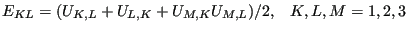(2)

where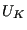are the displacement components in the material frame of reference and repeated indices imply summation over the appropriate range. In a linear analysis, this reduces to the familiar form: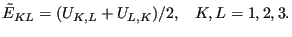(3)

The Eulerian strain satisfies ():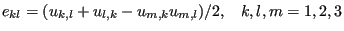(4)

where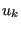are the displacements components in the spatial frame of reference.

Finally, the deviatoric elastic left Cauchy-Green tensor is defined by ():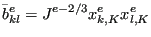(5)

where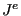is the elastic Jacobian and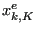is the elastic deformation gradient. The above formulas apply for Cartesian coordinate systems.

The stress measure consistent with the Lagrangian strain is the second Piola-Kirchhoff stress S. This stress, which is internally used in CalculiX for all applications (the so-called total Lagrangian approach, see ), can be transformed into the first Piola-Kirchhoff stress P (the so-called engineering stress, a non-symmetric tensor) and into the Cauchy stress t (true stress). All CalculiX input (e.g. distributed loading) and output is in terms of true stress. In a tensile test on a specimen with length L the three stress measures are related by: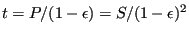(6)

where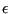is the engineering strain defined by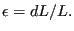(7)

The treatment of the thermal strain depends on whether the analysis is geometrically linear or nonlinear. For isotropic material the thermal strain tensor amounts to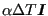, where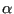is the expansion coefficient,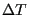is the temperature change since the initial state and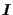is the second order identity tensor. For geometrically linear calculations the thermal strain is subtracted from the total strain to obtain the mechanical strain: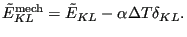(8)

In a nonlinear analysis the thermal strain is subtracted from the deformation gradient in order to obtain the mechanical deformation gradient. Indeed, assuming a multiplicative decomposition of the deformation gradient one can write: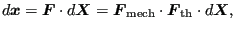(9)

where the total deformation gradient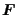is written as the product of the mechanical deformation gradient and the thermal deformation gradient. For isotropic materials the thermal deformation gradient can be written as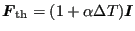and consequently: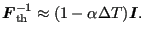(10)

Therefore one obtains: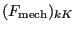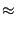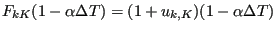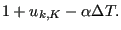(11)

Based on the mechanical deformation gradient the mechanical Lagrange strain is calculated and subsequently used in the material laws: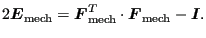(12)

Subsections